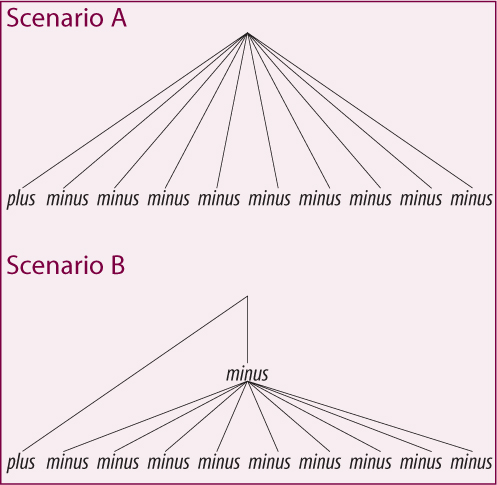Contributing Editor Xiao-Li Meng writes:

“How do you like your new job?” I keep getting asked. Like most jobs, mine has seen days I wished to forget before sunset and evenings I wanted to remember after sunrise. One of these evenings was a talk at our graduate student center, given by Professor Richard Tarrant, on “Editing Classical Latin Texts: Reflections of a Survivor.”

I attended it because I desperately need my General Education. Trained as a pure mathematician in college with the only “impure” elective course being “Mathematical Equations for Physics,” I have constantly embarrassed myself, especially during 57 admissions meetings, by not knowing which language goes with which country or which holy text belongs to which religion. I have become a living example of the importance of providing a T-shaped education, emphasizing deep scholarship and expertise as well as broad knowledge and skills.

Richard started by asking why there are so many editions of classical texts, such as Satyrica or Metamorphoses. The answer turns out to be one that we statisticians appreciate: uncertainty! The surviving copies of these texts may contain missing words, lost sections, scribal errors, misplaced segmentations, etc. These imperfections have left much for classicists to impute, infer, and interpret, and each edition may generate its own ambiguities for even more scholars to contemplate.

The problem truly belongs to statistical classics when one needs to infer an estimand, say a particular phrase in the original text, using data, the corresponding phrases given in various editions. Richard used a figure [below] to illustrate “a critical method” for classicists to accomplish such a task. In both scenarios, one manuscript shows the phrase being “plus”, but nine other manuscripts show “minus”. Obviously the odds of the original phrase being “minus” versus “plus” depend critically on whether the nine “minus” instances were independent interpretations (Scenario A) or they actually were all copies of an even earlier manuscript (Scenario B). A critical step for classicists is to establish which scenario occurred by often painstaking work, for example, demonstrating that any communications or collaborations among the nine scribes were inconceivable during their time.As it happens, another memorable evening in recent months reminded me of the importance of studying classical statistics. My student, Alex Blocker, and I have been working on building a theoretical foundation for preprocessing, which includes recalibration, normalization, compression, etc.; that is, anything done to the raw data before they are presented to the analyst. As the quality of such processing obviously matters, any statistical theory that ignores preprocessing is insufficient for addressing big data, where preprocessing is the norm rather than the exception.

Building such a theory turns out to be challenging. Consider a simple but realistic setting, where our scientific model is $P(X|θ)$, but we do not observe $X$ (e.g., the true gene expression). The raw data are $Y$ (e.g., intensity measurements for probes), which are subject to noise captured by an observation model $P(Y|X)$, free of $θ$. Often a preprocessor only has a guess of the scientific model $P(X|θ)$, denoted by $Q(X|η)$. Any complete theory of lossless compression then requires that we determine when a sufficient statistic $T(Y)$ for the $Y$-margin of the preprocessing model $P(Y|X)Q(X|η)$ will also be sufficient for the $Y$-margin of the (joint) scientific model $P(Y,X|θ)=P(Y|X)P(X|θ)$.

During our investigation, the following question extended an hour-long meeting from 5pm until midnight. Let $T=T(Y)$ and $S=S(X)$ be sufficient statistics respectively for $P(Y|θ)$ and $P(X|θ)$, the two margins of $P(Y, X|θ)=P(Y|X)P(X|θ)$. Clearly $S(X)$ is also sufficient for $P(Y, X|θ)$, and hence $P(Y|S, θ)=P(Y|S)$. As $S$ can be viewed as the parameter in this conditional model, we can ask when $T$ is also sufficient for $S$, that is, $P(Y|T, S)=P(Y|T)$? Evidently this is not true in general (e.g., when $S(X)=X$ ), and it is clearly true if $S$ is complete. But what if $S$ is minimally sufficient but not complete? (Completeness is often too strong a condition for our settings.)

Some have argued that the notion of completeness is out of date (though it is important for our investigation), but the concept of sufficiency must lie at the core of statistics or any branch of “Data Science”, even though the language may differ (e.g., lossless compression). We serve our students—and science—well when we teach both the contemporary variations and developments of (judiciously selected) classical concepts, and their well-established theoretical foundations. I therefore submit that a complete T-shaped PhD education in Statistics must require enough depth to activate students’ desires to “treasure hunt” classical statistics for contemporary benefit, as well as adequate breadth to enable them to connect seemingly unrelated subjects such as statistical classics.

Given that IMS has unique advantages for this educational endeavor, may I suggest it as one of IMS’s emphases during this International Year of Statistics, and beyond?